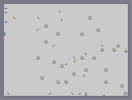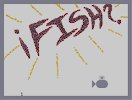### Rocky BalboaHover over the thumbnail for a full-size version.

Author toby3p0 author:toby3p0 n-art rated 2006-11-20 4 by 23 people. \$Rocky Balboa#toby3p0#none#00000000000000000000000000000000000000000000000000000000000000000000000000000000000000000000000000000000000000000000000000000000000000000000000000000000000000000000000000000000000000000000000000000000000000000000000000000000000000000000000000000000000000000000000000000000000000000000000000000000000000000000000000000000000000000000000000000000000000000000000000000000000000000000000000000000000000000000000000000000000000000000000000000000000000000000000000000000000000000000000000000000000000000000000000000000000000000000000000000000000000000000000000000000000000000000000000000000000000000000000000000000000000000000000000000000000000000000000000000000000000000000000000000000000000000000000000000000000000000|12^282,287!12^289,287!12^293,284!12^299,283!12^302,283!12^307,281!12^310,281!12^314,279!12^317,278!12^319,277!12^320,277!12^324,274!12^333,269!12^333,269!12^334,269!12^337,268!12^339,268!12^339,268!12^346,264!12^328,273!12^356,236!12^355,234!12^355,230!12^353,229!12^350,220!12^350,220!12^350,217!12^349,210!12^349,210!12^347,207!12^345,205!12^293,201!12^291,201!12^269,236!12^269,236!12^268,244!12^268,250!12^268,254!12^269,257!12^269,261!12^269,265!12^269,267!12^269,273!12^273,275!12^277,279!12^277,284!12^278,289!12^353,226!12^290,201!12^354,251!12^354,250!12^353,246!12^350,256!12^351,242!12^345,250!12^341,256!12^336,263!12^333,266!12^330,264!12^333,258!12^342,244!12^334,240!12^329,245!12^329,257!12^324,264!12^315,265!12^320,251!12^335,241!12^339,246!12^342,259!12^352,223!12^345,231!12^341,235!12^342,229!12^345,229!12^347,228!12^343,216!12^341,217!12^339,224!12^337,239!12^326,248!12^323,258!12^314,265!12^311,272!12^313,273!12^304,274!12^298,276!12^295,274!12^303,263!12^307,258!12^300,256!12^307,268!12^312,260!12^311,252!12^334,247!12^340,246!12^348,239!12^348,239!12^321,259!12^316,259!12^315,259!12^324,269!12^292,274!12^284,278!12^284,279!12^280,281!12^278,275!12^278,270!12^275,268!12^282,271!12^289,272!12^295,262!12^299,264!12^297,269!12^291,267!12^285,265!12^282,265!12^279,265!12^297,257!12^295,261!12^288,262!12^302,250!12^308,250!12^314,246!12^317,245!12^325,236!12^325,227!12^333,235!12^310,232!12^315,233!12^322,225!12^318,226!12^333,223!12^333,228!12^330,217!12^339,211!12^332,205!12^312,231!12^310,245!12^316,237!12^329,250!12^332,251!12^305,242!12^302,244!12^291,247!12^293,248!12^298,248!12^288,254!12^285,258!12^277,261!12^273,261!12^274,258!12^283,246!12^276,249!12^300,236!12^300,240!12^294,241!12^282,243!12^278,251!12^278,246!12^278,236!12^289,237!12^294,233!12^301,226!12^307,225!12^313,225!12^321,218!12^327,214!12^329,211!12^329,209!12^327,205!12^325,204!12^324,205!12^320,212!12^312,218!12^305,214!12^305,213!12^302,211!12^294,220!12^297,213!12^299,210!12^291,211!12^289,211!12^287,211!12^291,229!12^286,230!12^282,230!12^281,230!12^278,230!12^300,218!12^301,216!12^304,220!12^325,236!12^315,256!12^313,237!12^309,236!12^305,236!12^301,236!12^283,246!12^275,251!12^270,255!12^292,280!12^292,280!12^346,240!12^332,236!12^316,239!12^326,241!12^339,211!12^339,211!12^355,239!12^355,240!12^356,246!12^356,251!12^362,248!12^363,248!12^363,244!12^363,242!12^360,234!12^359,233!12^265,262!12^264,260!12^262,256!12^263,250!12^267,250!12^359,238!12^268,240!12^266,245!12^360,253!12^361,253!12^361,256!12^299,227!12^301,228!12^303,230!12^294,239!12^285,241!12^282,241!12^288,248!12^286,257!12^281,262!12^281,255!12^284,249!12^320,271!12^319,271!12^323,241!12^323,241!12^324,234!12^347,217!12^343,211!12^342,209!12^340,205!12^336,207!12^336,211!12^330,221!12^362,261!12^358,267!12^350,275!12^349,275!12^348,275!12^345,278!12^344,279!12^340,282!12^339,282!12^335,284!12^331,286!12^330,286!12^327,287!12^323,288!12^316,288!12^311,288!12^308,288!12^306,288!12^303,287!12^365,256!12^366,255!12^368,255!12^370,256!12^376,256!12^380,257!12^380,257!12^384,257!12^385,257!12^385,258!12^386,261!12^389,263!12^389,266!12^390,270!12^390,278!12^390,282!12^388,288!12^382,293!12^376,300!12^376,300!12^372,301!12^366,311!12^363,316!12^358,319!12^349,327!12^344,330!12^343,330!12^339,330!12^335,332!12^328,334!12^324,335!12^316,336!12^308,337!12^299,337!12^294,337!12^288,337!12^286,337!12^283,337!12^281,337!12^281,337!12^281,332!12^281,330!12^281,327!12^281,324!12^281,320!12^281,318!12^281,316!12^281,316!12^281,316!12^281,310!12^281,306!12^281,305!12^283,303!12^284,301!12^285,300!12^285,297!12^286,296!12^287,295!12^288,290!12^289,289!12^366,308!12^368,306!12^353,324!12^319,335!12^312,337!12^303,338!12^380,296!12^387,291!12^390,273!12^378,255!12^382,254!12^384,254!12^387,253!12^388,253!12^391,253!12^395,253!12^396,255!12^396,260!12^398,262!12^399,264!12^400,265!12^401,268!12^401,274!12^402,278!12^403,281!12^403,286!12^403,292!12^403,297!12^400,302!12^397,308!12^393,311!12^393,311!12^391,312!12^388,320!12^386,325!12^382,330!12^376,338!12^375,340!12^370,346!12^369,346!12^361,354!12^355,356!12^351,358!12^348,359!12^343,361!12^333,364!12^327,364!12^325,363!12^322,363!12^311,365!12^305,365!12^303,365!12^296,365!12^288,365!12^288,365!12^283,365!12^277,365!12^275,365!12^270,360!12^267,357!12^266,354!12^266,348!12^266,345!12^269,340!12^271,340!12^274,338!12^277,333!12^390,316!12^379,336!12^372,342!12^366,350!12^316,363!12^291,365!12^339,363!12^406,270!12^406,270!12^409,271!12^411,275!12^413,279!12^414,280!12^415,281!12^419,283!12^422,285!12^422,287!12^424,289!12^426,290!12^426,290!12^426,292!12^426,294!12^426,298!12^426,303!12^426,304!12^426,308!12^426,315!12^426,317!12^426,322!12^426,327!12^426,330!12^426,335!12^426,337!12^425,340!12^423,348!12^422,351!12^421,354!12^421,357!12^418,367!12^414,375!12^413,376!12^411,381!12^408,386!12^404,392!12^403,392!12^394,397!12^387,399!12^379,399!12^369,399!12^363,399!12^358,399!12^357,398!12^343,399!12^338,399!12^337,399!12^329,396!12^324,393!12^322,393!12^314,393!12^308,393!12^303,391!12^298,387!12^293,385!12^286,380!12^286,379!12^280,373!12^279,370!12^350,399!12^399,392!12^418,361!12^425,343!12^292,326!12^292,308!12^304,307!12^304,323!12^317,316!12^308,303!12^323,298!12^321,315!12^329,318!12^336,312!12^327,304!12^323,304!12^343,292!12^341,291!12^340,306!12^353,306!12^353,298!12^354,286!12^365,289!12^378,281!12^373,267!12^330,300!12^329,300!12^343,313!12^337,316!12^324,321!12^312,319!12^305,314!12^309,303!12^305,294!12^299,295!12^290,305!12^285,321!12^295,321!12^298,322!12^301,327!12^306,327!12^321,327!12^292,357!12^293,357!12^304,357!12^310,357!12^315,357!12^324,357!12^332,357!12^340,355!12^345,351!12^352,347!12^354,344!12^360,344!12^365,344!12^371,341!12^352,349!12^342,353!12^325,356!12^311,357!12^295,357!12^288,361!12^283,362!12^280,359!12^280,355!12^287,351!12^295,347!12^310,343!12^334,350!12^313,349!12^336,345!12^349,339!12^352,336!12^349,343!12^340,340!12^345,333!12^358,333!12^367,330!12^369,328!12^369,325!12^367,322!12^376,322!12^380,309!12^390,301!12^304,377!12^308,377!12^315,377!12^317,377!12^326,377!12^339,376!12^350,371!12^358,365!12^361,364!12^361,364!12^368,364!12^376,358!12^381,355!12^378,371!12^370,377!12^365,380!12^354,381!12^346,381!12^335,381!12^322,381!12^313,381!12^310,378!12^325,376!12^335,376!12^349,370!12^359,371!12^363,373!12^367,370!12^376,362!12^380,359!12^369,373!12^357,374!12^348,375!12^344,373!12^316,371!12^308,369!12^294,369!12^284,376!12^293,380!12^303,380!12^311,384!12^336,384!12^340,387!12^341,389!12^353,387!12^356,392!12^369,393!12^362,385!12^384,383!12^387,380!12^375,375!12^379,388!12^386,388!12^392,381!12^380,369!12^382,361!12^394,365!12^394,369!12^402,373!12^414,368!12^404,360!12^390,355!12^385,358!12^383,349!12^384,345!12^395,346!12^404,354!12^414,351!12^408,341!12^393,333!12^392,328!12^407,324!12^414,318!12^408,316!12^411,306!12^412,304!12^414,287!12^417,340!12^395,327!12^417,331!12^402,339!12^296,365!12^296,367!12^298,365!12^305,365!12^313,365!12^317,365!12^326,365!12^335,365!12^344,362!12^357,356!12^362,351!12^369,344!12^369,344!12^378,336!12^380,397!12^377,398!12^371,398!12^371,398!12^401,394!12^401,394!12^424,306!12^424,308!12^424,312!12^329,392!12^322,392!12^318,393!12^312,393!12^305,393!12^301,391!12^295,387!12^288,379!12^280,373!12^275,347!12^268,356!12^265,362!12^263,366!12^262,366!12^257,370!12^256,370!12^251,374!12^251,374!12^193,441!12^194,443!12^195,443!12^196,450!12^197,450!12^202,457!12^200,457!12^197,458!12^194,458!12^192,458!12^188,460!12^188,461!12^188,461!12^188,462!12^183,469!12^183,473!12^182,480!12^181,482!12^180,482!12^174,490!12^170,498!12^168,499!12^165,506!12^165,507!12^165,513!12^169,499!12^175,482!12^175,484!12^175,490!12^170,495!12^196,453!12^204,467!12^202,467!12^198,468!12^196,471!12^195,471!12^195,472!12^194,475!12^194,477!12^194,481!12^194,483!12^194,487!12^196,489!12^197,490!12^200,493!12^203,494!12^206,496!12^208,497!12^215,498!12^218,498!12^224,498!12^229,498!12^233,498!12^186,482!12^186,482!12^186,488!12^186,490!12^188,494!12^194,499!12^198,503!12^198,503!12^199,504!12^202,507!12^203,507!12^206,507!12^211,509!12^211,509!12^213,510!12^216,510!12^219,512!12^221,513!12^223,513!12^226,513!12^230,513!12^232,513!12^233,513!12^239,513!12^203,465!12^203,465!12^203,463!12^203,461!12^203,459!12^199,453!12^203,485!12^201,472!12^201,471!12^203,481!12^202,475!12^162,517!12^160,520!12^159,522!12^157,527!12^156,532!12^155,538!12^155,544!12^157,546!12^160,548!12^165,549!12^172,552!12^177,556!12^180,560!12^184,564!12^190,568!12^195,568!12^204,564!12^200,567!12^210,563!12^215,560!12^219,559!12^224,554!12^231,551!12^230,552!12^224,556!12^235,550!12^237,548!12^240,546!12^243,543!12^246,541!12^226,486!12^226,488!12^226,488!12^228,488!12^229,494!12^233,499!12^235,500!12^236,504!12^237,506!12^237,509!12^237,511!12^239,513!12^242,515!12^242,516!12^242,517!12^242,519!12^243,523!12^244,524!12^245,527!12^246,527!12^248,527!12^251,528!12^254,528!12^258,528!12^260,528!12^262,529!12^264,529!12^266,529!12^270,529!12^272,529!12^276,529!12^278,529!12^280,528!12^283,527!12^289,525!12^292,522!12^294,520!12^297,517!12^300,515!12^300,513!12^300,511!12^300,508!12^251,541!12^251,541!12^252,540!12^254,538!12^254,537!12^254,535!12^254,534!12^254,532!12^255,537!12^255,541!12^255,542!12^255,546!12^257,547!12^259,551!12^260,554!12^261,555!12^263,555!12^253,550!12^252,551!12^250,557!12^250,557!12^248,562!12^248,564!12^248,567!12^248,571!12^248,572!12^248,574!12^268,247!12^267,245!12^263,245!12^262,245!12^261,240!12^261,240!12^261,244!12^261,246!12^261,240!12^261,237!12^261,232!12^261,231!12^261,231!12^260,223!12^260,220!12^260,214!12^261,212!12^262,211!12^262,210!12^264,203!12^264,202!12^265,197!12^268,194!12^270,193!12^272,190!12^273,189!12^275,185!12^281,184!12^287,183!12^292,181!12^292,180!12^301,180!12^309,180!12^315,180!12^321,180!12^326,180!12^332,180!12^338,181!12^341,184!12^346,190!12^348,192!12^349,196!12^352,203!12^353,210!12^354,216!12^356,223!12^358,228!12^276,238!12^274,240!12^288,223!12^293,224!12^289,225!12^278,226!12^270,224!12^270,230!12^274,233!12^275,229!12^271,224!12^269,218!12^272,211!12^276,211!12^278,207!12^287,206!12^282,203!12^271,202!12^267,208!12^265,226!12^275,202!12^275,201!12^286,193!12^289,192!12^289,192!12^283,196!12^279,199!12^291,191!12^296,190!12^305,190!12^307,193!12^317,196!12^317,199!12^310,202!12^310,202!12^305,209!12^304,210!12^316,202!12^315,199!12^306,195!12^301,190!12^302,184!12^297,183!12^298,186!12^299,187!12^291,187!12^314,185!12^314,186!12^314,187!12^320,191!12^328,188!12^334,188!12^341,196!12^342,196!12^349,200!12^343,195!12^339,191!12^335,188!12^330,185!12^322,185!12^322,187!12^334,196!12^344,197!12^337,206!12^331,195!12^329,192!12^332,242!12^342,248!12^345,249!12^345,249!12^338,257!12^322,252!12^335,217!12^344,222!12^346,247!12^336,233!12^292,257!12^349,314!12^316,310!12^314,310!12^313,305!12^295,309!12^295,310!12^276,546!12^278,546!12^282,546!12^287,546!12^291,546!12^299,546!12^304,546!12^309,546!12^313,546!12^319,546!12^324,546!12^327,545!12^336,544!12^340,544!12^346,544!12^350,544!12^358,543!12^364,540!12^369,537!12^368,536!12^372,534!12^376,531!12^378,528!12^381,524!12^383,522!12^391,513!12^391,512!12^391,509!12^388,519!12^386,519!12^396,504!12^397,503!12^397,499!12^403,492!12^409,488!12^411,485!12^416,479!12^430,467!12^430,467!12^431,464!12^436,460!12^440,458!12^441,455!12^446,450!12^452,446!12^455,444!12^457,441!12^465,435!12^466,432!12^472,425!12^475,422!12^477,418!12^461,437!12^423,471!12^422,473!12^418,479!12^330,545!12^400,495!12^469,429!12^479,413!12^480,408!12^481,402!12^481,401!12^482,399!12^484,392!12^484,391!12^485,391!12^485,386!12^486,378!12^486,379!12^488,373!12^488,370!12^492,352!12^493,351!12^493,349!12^493,345!12^490,351!12^490,357!12^490,359!12^490,369!12^492,362!12^494,339!12^494,337!12^494,336!12^495,335!12^495,332!12^495,329!12^495,323!12^496,320!12^497,319!12^497,317!12^497,314!12^497,313!12^498,307!12^499,302!12^500,299!12^501,296!12^501,295!12^501,291!12^501,285!12^503,279!12^506,272!12^507,271!12^506,275!12^506,266!12^507,264!12^508,262!12^508,261!12^505,258!12^505,256!12^505,255!12^500,251!12^494,247!12^491,247!12^489,247!12^486,247!12^482,247!12^478,247!12^478,247!12^470,245!12^466,245!12^462,245!12^456,243!12^452,240!12^446,239!12^445,239!12^443,237!12^436,236!12^432,235!12^428,234!12^421,234!12^417,234!12^414,233!12^409,234!12^405,236!12^401,238!12^398,239!12^394,242!12^392,246!12^390,251!12^410,229!12^415,228!12^416,227!12^417,224!12^421,221!12^424,219!12^426,218!12^430,215!12^434,213!12^439,210!12^443,206!12^448,201!12^452,194!12^454,191!12^457,186!12^458,183!12^457,182!12^453,178!12^453,174!12^455,169!12^458,166!12^464,163!12^466,160!12^468,158!12^472,154!12^476,150!12^479,145!12^479,143!12^481,140!12^481,137!12^481,131!12^487,125!12^492,119!12^492,113!12^493,109!12^498,103!12^498,101!12^500,96!12^504,89!12^507,81!12^508,75!12^510,69!12^510,67!12^513,64!12^514,64!12^517,64!12^522,63!12^526,62!12^531,61!12^535,61!12^540,61!12^544,61!12^545,62!12^547,62!12^551,62!12^557,67!12^558,65!12^560,62!12^560,60!12^560,57!12^558,56!12^558,54!12^556,44!12^556,40!12^554,39!12^550,38!12^547,38!12^541,38!12^534,38!12^529,38!12^524,38!12^522,38!12^519,40!12^516,42!12^516,46!12^516,49!12^516,51!12^514,59!12^512,60!12^520,34!12^520,33!12^518,29!12^517,27!12^555,38!12^555,37!12^556,30!12^557,29!12^557,26!12^557,25!12^516,27!12^512,30!12^509,30!12^505,30!12^502,30!12^498,30!12^497,28!12^501,28!12^505,27!12^507,25!12^510,25!12^557,47!12^555,67!12^555,67!12^555,69!12^555,69!12^555,72!12^555,77!12^555,82!12^555,85!12^555,88!12^555,94!12^555,100!12^555,101!12^555,107!12^552,117!12^550,124!12^550,124!12^550,125!12^550,126!12^549,131!12^547,136!12^547,140!12^547,146!12^547,153!12^547,156!12^547,161!12^547,161!12^547,166!12^547,172!12^546,177!12^546,183!12^544,189!12^543,190!12^540,199!12^540,199!12^536,209!12^535,209!12^531,218!12^527,220!12^525,223!12^519,230!12^519,230!12^517,234!12^513,244!12^508,249!12^507,250!12^504,253!12^515,238!12^519,228!12^511,247!12^522,225!12^533,212!12^539,204!12^542,196!12^550,119!12^553,114!12^457,180!12^461,180!12^465,180!12^466,180!12^470,180!12^474,180!12^480,181!12^482,181!12^487,181!12^489,182!12^492,182!12^498,182!12^500,184!12^503,184!12^506,184!12^506,185!12^508,188!12^508,188!12^509,188!12^509,190!12^517,197!12^517,197!12^522,207!12^521,204!12^508,186!12^528,212!12^489,188!12^503,192!12^477,189!12^486,167!12^497,167!12^506,164!12^515,159!12^524,156!12^528,151!12^536,158!12^531,164!12^504,170!12^494,171!12^524,158!12^525,161!12^511,162!12^494,135!12^494,131!12^495,128!12^502,123!12^450,200!12^450,201!12^458,203!12^459,193!12^460,193!12^464,193!12^489,129!12^490,125!12^494,123!12^498,121!12^412,271!12^418,275!12^419,278!12^426,280!12^431,284!12^433,285!12^437,285!12^438,285!12^454,286!12^460,288!12^466,294!12^470,301!12^475,305!12^479,314!12^473,323!12^466,321!12^451,311!12^443,308!12^440,305!12^436,301!12^433,301!12^435,291!12^437,288!12^439,289!12^439,298!12^435,298!12^439,290!12^444,291!12^444,300!12^444,303!12^457,303!12^464,303!12^467,313!12^467,315!12^459,309!12^454,297!12^453,296!12^449,290!12^446,290!12^424,267!12^431,267!12^462,260!12^454,254!12^461,262!12^430,253!12^415,253!12^423,257!12^443,270!12^464,233!12^459,228!12^450,226!12^502,147!12^511,134!12^520,136!12^536,126!12^513,109!12^512,96!12^515,94!12^509,104!12^490,149!12^513,179!12^487,160!12^463,173!12^512,148!12^494,144!12^520,115!12^519,86!12^453,232!12^473,226!12^481,239!12^422,224!12^428,224!12^441,228!12^442,230!12^428,230!12^477,236!12^476,226!12^525,153!12^532,162!12^516,172!12^510,168!12^499,157!12^504,153!12^523,147!12^520,179!12^528,187!12^486,260!12^453,253!12^428,241!12^418,247!12^498,264!12^404,250!12^422,280!12^430,284!12^438,285!12^449,285!12^454,285!12^459,288!12^462,292!12^467,297!12^470,300!12^471,304!12^472,307!12^475,310!12^478,314!12^467,281!12^440,276!12^427,270!12^424,267!12^446,276!12^223,502!12^226,506!12^215,506!12^204,500!12^224,504!12^236,539!12^245,529!12^236,538!12^222,519!12^218,527!12^222,539!12^221,546!12^231,539!12^224,547!12^219,552!12^211,553!12^209,543!12^204,517!12^201,532!12^195,539!12^196,553!12^202,543!12^208,524!12^192,531!12^189,547!12^186,551!12^183,553!12^178,546!12^182,536!12^185,520!12^189,514!12^198,510!12^188,507!12^173,534!12^236,532!12^236,525!12^233,521!12^228,518!12^210,550!12^191,562!12^189,562!12^189,555!12^199,541!12^211,543!12^211,519!12^213,511!12^482,388!12^483,393!12^483,398!12^483,403!12^483,412!12^483,419!12^483,425!12^483,428!12^483,430!12^483,433!12^483,435!12^483,435!12^483,442!12^483,449!12^483,459!12^483,462!12^483,468!12^483,472!12^483,476!12^480,485!12^478,491!12^477,496!12^475,500!12^474,504!12^473,510!12^473,513!12^473,519!12^473,525!12^471,537!12^469,540!12^465,548!12^457,556!12^455,558!12^448,566!12^461,554!12^458,554!12^460,550!12^471,539!12^473,533!12^473,531!12^480,481!12^482,449!12^482,452!12^482,455!12^467,545!12^470,544!12^472,545!12^472,546!12^472,547!12^473,549!12^473,549!12^474,551!12^475,553!12^475,553!12^475,558!12^475,559!12^473,563!12^473,564!12^468,571!12^468,571!12^468,571!12^463,575!12^451,562!12^476,567!12^476,567!12^476,573!12^476,573!12^474,575!12^470,575!12^467,570!12^470,570!12^423,484!12^428,479!12^404,501!12^403,502!12^428,482!12^411,500!12^426,479!12^409,497!12^398,512!12^403,502!12^391,519!12^387,519!12^416,483!12^430,467!12^433,465!12^428,471!12^420,481!12^412,489!12^411,491!12^404,502!12^409,500!12^415,494!12^425,484!12^427,478!12^433,469!12^436,463!12^436,463!12^435,471!12^434,473!12^429,481!12^424,491!12^423,493!12^408,514!12^403,519!12^398,525!12^393,530!12^388,536!12^381,542!12^376,547!12^373,551!12^366,553!12^343,570!12^337,572!12^477,483!12^475,483!12^469,492!12^468,494!12^451,514!12^440,528!12^416,555!12^414,558!12^407,566!12^403,571!12^478,452!12^478,459!12^477,468!12^472,478!12^466,488!12^466,488!12^457,497!12^450,509!12^408,568!12^400,575!12^419,533!12^411,544!12^409,546!12^403,553!12^397,558!12^393,562!12^392,563!12^384,567!12^379,571!12^379,571!12^370,577!12^522,30!12^528,30!12^534,30!12^540,30!12^546,30!12^552,30!12^552,24!12^546,24!12^540,24!12^534,24!12^528,24!12^522,24!12^528,36!12^534,36!12^540,36!12^546,36!12^552,36!12^548,34!12^537,34!12^532,34!12^531,28!12^539,28!12^546,27!12^524,48!12^530,48!12^539,48!12^546,49!12^554,54!12^527,56!12^548,45!12^522,51!12^517,67!12^521,56!12^514,57!12^480,205!12^498,221!12^449,212!12^470,187!12^457,194!12^458,194!12^462,194!12^464,194!12^458,204!12^450,201!12^445,203!12^438,340!12^450,364!12^471,368!12^473,362!12^467,350!12^444,328!12^433,338!12^438,343!12^439,343!12^441,352!12^448,360!12^456,362!12^431,351!12^429,351!12^436,369!12^442,352!12^448,357!12^452,364!12^471,364!12^438,351!12^435,365!12^435,359!12^439,366!12^439,324!12^445,328!12^451,333!12^468,349!12^459,347!12^445,337!12^450,339!12^452,346!12^336,558!12^325,562!12^315,562!12^311,562!12^311,569!12^318,570!12^320,574!12^329,572!12^309,572!12^411,516!12^403,527!12^391,539!12^376,551!12^363,558!12^360,566!12^348,568!12^366,565!12^376,555!12^386,545!12^401,529!12^397,533!12^368,560!12^373,561!12^386,556!12^397,543!12^362,571!12^400,540!12^407,547!12^404,553!12^395,559!12^386,566!12^377,570!12^382,574!12^389,567!12^402,559!12^414,549!12^472,486!12^452,522!12^451,527!12^443,530!12^443,539!12^435,537!12^434,553!12^437,543!12^408,571!12^415,573!12^422,567!12^428,558!12^442,546!12^456,528!12^460,513!12^465,511!12^459,523!12^415,556!12^403,573!12^399,577!12^415,565!12^443,536!12^450,522!12^456,509!12^470,492!12^234,515!12^232,517!12^224,524!12^222,527!12^231,522!12^236,518!12^240,518!12^231,521!12^220,524!12^232,520!12^237,520!12^237,522!12^463,571!12^455,571!12^453,574!12^451,575!12^458,575!12^446,575!12^426,388!12^444,422!12^415,397!12^426,395!12^443,431!12^424,388!12^436,395!12^423,395!12^462,416!12^415,398!12^421,430!12^405,419!12^418,435!12^404,430!12^397,463!12^356,469!12^265,424!12^249,400!12^229,415!12^238,418!12^241,407!12^223,427!12^235,443!12^267,489!12^255,490!12^226,456!12^300,474!12^308,477!12^276,454!12^414,461!12^366,498!12^339,466!12^300,428!12^293,434!12^324,493!12^311,498!12^248,432!12^271,444!12^293,491!12^290,496!12^275,505!12^259,495!12^226,446!12^282,486!12^269,485!12^256,470!12^271,465!12^283,493!12^246,459!12^233,454!12^266,444!12^283,483!12^266,486!12^292,483!12^229,439!12^223,422!12^333,456!12^330,432!12^355,445!12^328,440!12^332,438!12^345,464!12^296,488!12^270,474!12^242,427!12^232,430!12^230,434!12^264,463!12^275,471!12^274,478!12^242,455!12^240,431!12^235,438!12^231,404!12^263,392!12^257,416!12^473,547!12^470,542!12^470,535!12^493,359!12^493,341!12^194,468!12^193,477!12^194,482!12^197,487!12^200,491!12^206,495!12^212,495!12^222,495!12^230,495!12^236,518!12^232,516!12^365,248!12^366,244!12^366,240!12^366,238!12^366,235!12^366,233!12^364,231!12^363,230!12^359,226!12^359,221!12^359,214!12^359,208!12^356,197!12^354,195!12^351,192!12^344,186!12^340,183!12^357,201!12^357,207!12^345,187!12^342,183!12^350,188!12^344,179!12^341,178!12^335,173!12^332,172!12^325,171!12^319,171!12^315,171!12^311,171!12^298,173!12^292,175!12^287,176!12^279,184!12^275,185!12^303,175!12^309,173!12^313,173!12^330,177!12^305,169!12^302,170!12^298,170!12^292,172!12^287,173!12^281,179!12^278,180!12^269,186!12^259,247!12^258,241!12^253,235!12^252,232!12^252,227!12^253,224!12^253,211!12^253,206!12^254,205!12^258,194!12^261,190!12^261,189!12^267,182!12^276,179!12^266,190!12^260,196!12^257,200!12^257,209!12^256,215!12^253,220!12^250,230!12^251,237!12^252,229!12^254,242!12^255,242!12^257,250!12^259,258!12^254,252!12^250,240!12^248,232!12^248,226!12^248,218!12^248,209!12^252,202!12^252,201!12^249,207!12^247,213!12^247,216!12^247,221!12^247,227!12^381,449!12^341,432!12^330,432!12^331,433!12^283,378!12^279,378!12^275,380!12^272,381!12^268,383!12^263,385!12^260,388!12^258,389!12^251,395!12^250,395!12^243,400!12^239,404!12^236,407!12^235,409!12^230,415!12^227,420!12^226,422!12^224,428!12^223,434!12^223,438!12^223,442!12^223,446!12^223,447!12^221,454!12^220,459!12^219,463!12^215,469!12^215,470!12^212,472!12^208,475!12^204,479!12^213,468!12^214,465!12^216,461!12^219,454!12^220,446!12^221,437!12^222,425!12^222,424!12^228,418!12^231,412!12^235,407!12^240,402!12^247,397!12^251,392!12^255,389!12^262,386!12^272,381!12^272,381!12^279,381!12^247,377!12^245,378!12^242,380!12^240,380!12^237,381!12^233,384!12^229,387!12^226,388!12^222,392!12^220,399!12^218,402!12^214,405!12^212,408!12^210,411!12^205,417!12^200,419!12^196,422!12^194,426!12^192,432!12^192,435!12^192,439!12^207,414!12^221,392!12^226,386!12^224,390!12^205,435!12^207,442!12^212,442!12^219,425!12^209,424!12^218,410!12^223,401!12^224,398!12^225,400!12^217,415!12^209,423!12^202,427!12^202,432!12^207,435!12^218,427!12^241,387!12^261,376!12^210,460!12^500,23!12^346,256!12^306,277!12^300,268!12^320,232!12^329,232!12^349,235!12^307,263!12^272,245!12^284,235!12^256,227!12^316,246!12^305,255!12^295,256!12^291,249!12^307,248!12^337,250!12^339,250!12^343,247!12^343,234!12^342,228!12^327,233!12^326,235!12^319,247!12^313,256!12^317,264!12^333,266!12^343,265!12^345,263!12^349,258!12^348,244!12^343,242!12^302,269!12^296,277!12^290,280!12^287,280!12^285,271!12^283,257!12^276,248!12^327,218!12^321,212!12^316,211!12^313,211!12^311,210!12^309,211!12^309,218!12^315,218!12^318,215!12^315,205!12^306,207!12^297,208!12^289,211!12^284,220!12^276,228!12^263,231!12^262,229!12^277,227!12^283,220!12^283,214!12^285,211!12^297,207!12^302,199!12^303,197!12^314,197!12^317,197!12^324,202!12^332,203!12^335,203!12^342,203!12^334,202!12^288,216!12^278,218!12^275,218!12^271,218!12^266,218!12^265,220!12^261,221!12^281,225!12^306,225!12^307,221!12^326,220!12^331,222!12^325,225!12^313,219!12^303,220!12^290,231!12^294,239!12^309,245!12^310,251!12^329,254!12^331,198!12^320,198!12^298,202!12^296,202!12^294,202!12^295,199!12^299,196!12^317,192!12^323,192!12^326,195!12^334,200!12^254,200!12^259,191!12^260,190!12^262,186!12^266,181!12^268,178!12^272,174!12^281,171!12^286,171!12^292,171!12^299,171!12^300,170!12^310,169!12^316,169!12^324,169!12^335,170!12^339,171!12^344,176!12^347,182!12^347,184!12^349,188!12^276,172!12^273,172!12^362,268!12^320,461!12^309,468!12^295,458!12^282,445!12^270,434!12^297,443!12^297,450!12^281,429!12^387,415!12^417,435!12^403,445!12^402,445!12^350,420!12^332,411!12^383,474!12^398,446!12^399,418!12^366,493!12^351,495!12^344,495!12^331,498!12^320,508!12^318,517!12^326,503!12^345,494!12^364,494!12^371,496!12^364,508!12^364,495!12^378,507!12^364,529!12^328,538!12^385,504!12^318,538!12^267,571!12^277,564!12^277,562!12^277,571!12^266,570!12^298,575!12^281,571!12^265,575!12^295,566!12^253,434!12^269,435!12^281,445!12^295,457!12^267,443!12^269,445!12^255,486!12^226,476!12^275,497!12^316,463!12^272,422!12^301,418!12^318,414!12^275,402!12^280,414!12^283,439!12^287,445!12^275,433!12^384,451!12^361,442!12^341,428!12^369,461!12^366,468!12^339,454!12^331,447!12^356,467!12^254,457!12^274,465!12^276,465!12^266,449!12^277,455!12^277,459!12^169,526!12^179,504!12^459,472!12^460,455!12^447,480!12^443,465!12^452,467!12^434,503!12^438,499!12^447,493!12^448,492!12^429,506!12^427,522!12^301,528!12^300,528!12^308,515!12^397,467!12^383,473!12^391,468!12^417,445!12^426,427!12^344,404!12^298,410!12^400,414!12^348,418!12^348,418!12^375,420!12^395,426!12^371,424!12^387,407!12^393,430!12^394,428!12^515,215!12^507,233!12^541,144!12^527,125!12^513,124!12^496,117!12^509,98!12^507,86!12^517,68!12^534,98!12^537,82!12^507,86!12^498,109!12^506,112!12^529,86!12^546,75!12^546,103!12^537,162!12^531,192!12^492,253!12^466,193!12^506,210!12^466,213!12^483,217!12^507,206!12^485,221!12^452,322!12^471,351!12^441,437!12^313,496!12^242,416!12^311,362!12^386,397!12^429,428!12^481,327!12^462,310!12^395,364!12^308,513!12^287,496!12^319,400!12^319,401!12^288,400!12^280,395!12^276,404!12^334,433!12^370,463!12^249,489!12^234,494!12^266,517!12^298,516!12^356,515!12^432,454!12^475,397!12^446,392!12^335,455!12^296,438!12^314,420!12^295,408!12^249,516!12^310,513!12^264,501!12^255,503!12^385,491!12^318,489!12^305,471!12^303,462!12^306,449!12^465,391!12^473,354!12^480,346!12^481,340!12^475,339!12^459,327!12^473,374!12^481,570!12^481,566!12^481,560!12^481,556!12^477,552!12^476,547!12^476,542!12^477,536!12^477,534!12^477,525!12^477,521!12^476,515!12^478,506!12^479,500!12^480,574!12^476,508!12^476,503!12^479,487!12^479,494!12^479,489!12^480,483!12^484,472!12^486,463!12^481,474!12^479,479!12^481,467!12^481,462!12^485,459!12^485,455!12^486,440!12^486,438!12^483,433!12^482,424!12^482,422!12^482,399!12^484,397!12^484,407!12^483,414!12^488,379!12^490,374!12^491,368!12^490,368!12^488,368!12^492,365!12^492,361!12^493,354!12^492,351!12^491,351!12^493,346!12^493,334!12^494,331!12^496,329!12^498,325!12^498,322!12^497,321!12^497,318!12^497,310!12^498,301!12^500,293!12^505,282!12^505,284!12^506,269!12^507,269!12^507,264!12^507,261!12^507,260!12^511,249!12^511,249!12^514,245!12^517,241!12^518,237!12^522,230!12^522,230!12^529,221!12^531,217!12^532,212!12^533,207!12^536,204!12^540,198!12^543,190!12^546,182!12^546,179!12^546,165!12^546,164!12^546,160!12^545,156!12^544,153!12^547,150!12^549,148!12^546,143!12^546,143!12^548,136!12^552,127!12^549,117!12^549,116!12^551,112!12^552,106!12^555,87!12^555,87!12^555,87!12^555,74!12^555,73!12^553,66!12^550,155!12^549,155!12^549,153!12^549,149!12^549,148!12^549,146!12^549,141!12^548,139!12^548,136!12^549,161!12^549,166!12^549,168!12^549,174!12^548,177!12^546,182!12^544,184!12^514,53!12^513,55!12^509,66!12^508,72!12^508,78!12^504,88!12^503,92!12^502,95!12^499,101!12^494,109!12^491,115!12^487,123!12^485,126!12^483,130!12^480,133!12^478,137!12^477,140!12^477,150!12^476,154!12^472,156!12^468,158!12^463,161!12^457,167!12^456,173!12^456,181!12^456,185!12^449,195!12^445,202!12^439,208!12^435,213!12^426,220!12^417,226!12^412,230!12^399,236!12^394,239!12^388,246!12^386,250!12^383,254!12^383,253!12^376,253!12^371,252!12^368,246!12^368,243!12^368,241!12^368,240!12^369,234!12^367,229!12^365,227!12^360,218!12^359,217!12^359,212!12^359,211!12^359,205!12^358,199!12^356,196!12^351,192!12^350,187!12^348,183!12^346,179!12^338,170!12^336,168!12^335,168!12^331,168!12^327,167!12^326,166!12^326,165!12^320,164!12^315,163!12^311,163!12^308,164!12^304,165!12^300,165!12^295,165!12^294,165!12^287,166!12^281,168!12^278,170!12^275,171!12^272,172!12^268,174!12^264,177!12^261,181!12^259,184!12^259,184!12^254,192!12^252,196!12^249,208!12^249,209!12^248,216!12^248,224!12^248,229!12^248,235!12^250,239!12^251,242!12^253,249!12^255,252!12^257,261!12^263,266!12^267,266!12^268,268!12^275,275!12^274,282!12^277,285!12^281,289!12^283,294!12^281,304!12^279,305!12^278,311!12^278,315!12^278,320!12^278,321!12^277,331!12^275,338!12^271,343!12^270,344!12^268,344!12^265,342!12^262,350!12^264,353!12^264,358!12^258,365!12^255,365!12^249,371!12^241,373!12^238,376!12^226,387!12^223,390!12^226,382!12^230,378!12^234,374!12^242,371!12^247,368!12^254,364!12^262,361!12^253,366!12^241,373!12^231,381!12^224,383!12^220,392!12^217,396!12^214,402!12^209,406!12^205,410!12^203,412!12^201,413!12^198,417!12^194,421!12^191,426!12^190,434!12^191,439!12^192,444!12^192,444!12^191,452!12^191,456!12^183,465!12^182,467!12^181,472!12^181,473!12^178,483!12^173,482!12^168,487!12^167,491!12^162,500!12^160,504!12^160,504!12^160,511!12^160,514!12^160,518!12^159,522!12^156,525!12^152,530!12^152,533!12^152,536!12^152,543!12^153,547!12^155,548!12^163,553!12^164,553!12^166,553!12^169,554!12^171,556!12^174,556!12^177,559!12^180,561!12^184,562!12^187,563!12^190,567!12^193,568!12^195,568!12^199,568!12^205,568!12^207,567!12^213,564!12^217,563!12^220,562!12^221,562!12^232,556!12^234,552!12^235,552!12^241,548!12^244,546!12^247,546!12^250,546!12^241,556!12^223,562!12^328,280!12^333,279!12^327,281!12^320,283!12^316,283!12^313,283!12^309,283!12^322,281!12^329,281!12^334,281!12^337,281!12^342,281!12^344,279!12^345,277!12^346,275!12^352,269!12^353,269!12^355,269!12^360,267!12^362,263!12^362,260!12^363,274!12^356,276!12^381,271!12^368,287!12^359,295!12^352,305!12^402,269!12^402,273!12^402,281!12^402,285!12^402,295!12^401,300!12^400,304!12^396,312!12^392,319!12^390,324!12^386,330!12^381,335!12^376,339!12^371,346!12^366,350!12^358,354!12^353,359!12^347,361!12^339,363!12^339,363!12^327,365!12^317,366!12^309,366!12^305,366!12^303,366!12^288,367!12^283,367!12^276,367!12^271,367!12^268,364!12^266,362!12^265,359!12^48,60!12^48,48!12^48,54!12^48,42!12^48,36!12^48,30!12^54,30!12^60,30!12^66,30!12^72,30!12^72,30!12^84,30!12^78,30!12^90,30!12^96,30!12^102,30!12^108,30!12^114,36!12^120,36!12^126,42!12^132,48!12^132,54!12^138,66!12^48,66!12^48,72!12^48,78!12^48,84!12^48,90!12^48,96!12^48,108!12^48,102!12^48,114!12^48,120!12^48,126!12^48,132!12^48,138!12^48,144!12^54,144!12^60,144!12^66,144!12^72,144!12^78,144!12^78,138!12^78,132!12^78,126!12^78,120!12^78,114!12^84,114!12^90,114!12^90,120!12^96,126!12^96,132!12^102,138!12^102,144!12^138,144!12^132,144!12^126,144!12^120,144!12^114,144!12^108,144!12^126,114!12^126,120!12^132,126!12^132,132!12^138,138!12^132,72!12^132,78!12^126,84!12^120,90!12^114,96!12^120,108!12^78,72!12^78,66!12^78,60!12^78,54!12^84,54!12^90,54!12^96,54!12^102,60!12^102,66!12^96,72!12^90,72!12^84,72!12^192,144!12^198,144!12^204,144!12^210,144!12^186,138!12^174,132!12^168,132!12^162,126!12^180,138!12^240,126!12^234,132!12^228,132!12^222,138!12^216,138!12^246,120!12^246,114!12^156,120!12^156,114!12^150,108!12^150,102!12^252,108!12^252,102!12^144,96!12^144,90!12^144,84!12^144,78!12^258,96!12^258,90!12^258,84!12^258,78!12^150,72!12^150,66!12^156,60!12^156,54!12^162,48!12^168,42!12^174,42!12^180,36!12^186,36!12^192,30!12^198,30!12^204,30!12^210,30!12^216,36!12^222,36!12^228,42!12^234,42!12^240,48!12^246,54!12^246,60!12^252,66!12^252,72!12^198,108!12^204,108!12^192,102!12^192,96!12^192,84!12^192,78!12^192,72!12^192,90!12^210,102!12^210,96!12^210,90!12^210,84!12^210,78!12^210,72!12^204,66!12^198,66!12^138,60!12^120,102!12^264,78!12^264,84!12^264,90!12^264,96!12^270,102!12^270,108!12^276,114!12^276,120!12^282,126!12^288,132!12^294,132!12^300,138!12^306,138!12^312,144!12^318,144!12^324,144!12^330,144!12^336,138!12^342,138!12^348,132!12^354,132!12^360,126!12^366,120!12^366,114!12^270,72!12^270,66!12^276,60!12^276,54!12^282,48!12^288,42!12^294,42!12^300,36!12^306,36!12^312,30!12^318,30!12^324,30!12^330,30!12^336,36!12^342,36!12^348,42!12^354,42!12^360,48!12^366,54!12^366,60!12^372,66!12^372,72!12^372,108!12^372,102!12^366,102!12^360,102!12^354,102!12^366,72!12^360,72!12^354,72!12^348,72!12^342,72!12^348,102!12^342,102!12^336,72!12^336,102!12^330,102!12^330,72!12^330,66!12^306,72!12^306,66!12^306,78!12^306,84!12^306,96!12^306,90!12^306,102!12^306,108!12^330,108!12^312,60!12^318,60!12^324,60!12^312,114!12^318,114!12^324,114!12^378,30!12^378,36!12^378,42!12^378,48!12^378,54!12^378,66!12^378,60!12^378,72!12^378,78!12^378,84!12^378,90!12^378,96!12^378,102!12^378,108!12^378,114!12^378,120!12^378,126!12^378,132!12^378,138!12^378,144!12^384,144!12^390,144!12^396,144!12^384,30!12^390,30!12^396,30!12^402,30!12^408,30!12^402,144!12^408,144!12^414,30!12^414,36!12^414,42!12^414,48!12^414,54!12^420,54!12^426,48!12^432,42!12^438,36!12^444,30!12^450,30!12^456,30!12^462,30!12^468,30!12^474,30!12^474,138!12^414,144!12^414,138!12^426,120!12^432,126!12^438,132!12^444,138!12^450,144!12^456,144!12^462,144!12^468,144!12^474,144!12^414,132!12^414,126!12^414,60!12^420,120!12^420,114!12^480,30!12^486,30!12^480,132!12^474,126!12^480,36!12^474,42!12^468,48!12^462,54!12^456,60!12^450,66!12^444,72!12^438,78!12^432,84!12^468,120!12^462,114!12^456,108!12^450,102!12^444,96!12^438,90!12^492,30!12^492,36!12^498,42!12^498,48!12^504,54!12^504,60!12^510,60!12^588,30!12^582,30!12^576,30!12^594,30!12^600,30!12^606,30!12^570,30!12^570,36!12^564,42!12^564,48!12^558,48!12^558,54!12^606,36!12^600,42!12^600,48!12^594,54!12^594,60!12^588,66!12^588,72!12^582,78!12^582,84!12^576,90!12^576,96!12^570,102!12^570,108!12^570,114!12^570,126!12^570,120!12^570,132!12^570,138!12^570,144!12^564,144!12^558,144!12^552,144!12^348,300!12^115,33!12^115,33!12^115,33!12^120,35!12^126,39!12^128,41!12^131,46!12^131,46!12^132,53!12^132,54!12^133,58!12^133,61!12^133,62!12^132,66!12^130,72!12^128,77!12^128,80!12^124,85!12^120,89!12^117,92!12^116,95!12^116,99!12^118,101!12^118,107!12^120,113!12^123,115!12^124,120!12^130,124!12^130,125!12^131,133!12^134,138!12^137,141!12^137,140!12^137,137!12^135,135!12^134,131!12^130,126!12^127,122!12^125,116!12^125,116!12^124,111!12^122,108!12^120,105!12^117,102!12^101,141!12^101,136!12^100,134!12^98,132!12^98,131!12^95,129!12^93,128!12^91,124!12^90,121!12^88,118!12^87,117!12^86,117!12^137,70!12^136,71!12^135,61!12^135,57!12^135,55!12^135,52!12^146,89!12^146,86!12^146,80!12^146,74!12^148,70!12^152,66!12^152,63!12^155,57!12^160,53!12^161,49!12^163,46!12^166,43!12^168,41!12^173,38!12^178,37!12^182,34!12^189,32!12^192,32!12^196,31!12^201,31!12^203,29!12^209,30!12^215,31!12^219,32!12^224,36!12^228,37!12^229,37!12^237,42!12^240,48!12^244,51!12^246,54!12^247,57!12^248,59!12^250,62!12^250,64!12^256,73!12^259,79!12^259,83!12^259,88!12^259,95!12^257,96!12^255,101!12^254,104!12^252,107!12^252,107!12^242,124!12^240,124!12^236,131!12^235,131!12^232,135!12^227,136!12^224,138!12^222,139!12^219,140!12^214,141!12^209,143!12^204,143!12^199,143!12^191,143!12^184,143!12^180,140!12^178,137!12^174,135!12^170,133!12^166,129!12^161,125!12^159,122!12^157,120!12^154,116!12^151,112!12^149,107!12^147,108!12^146,102!12^145,98!12^144,96!12^144,90!12^258,94!12^257,100!12^253,108!12^252,109!12^250,114!12^247,119!12^246,119!12^241,48!12^237,45!12^235,44!12^232,39!12^228,36!12^225,35!12^97,72!12^98,72!12^100,72!12^100,67!12^100,62!12^100,60!12^98,58!12^98,57!12^96,56!12^93,54!12^88,54!12^83,54!12^80,54!12^78,57!12^76,60!12^76,62!12^77,65!12^77,69!12^77,73!12^80,73!12^84,73!12^87,73!12^90,73!12^95,73!12^95,73!12^111,34!12^110,34!12^103,32!12^99,32!12^94,32!12^88,32!12^79,32!12^74,32!12^68,32!12^68,32!12^61,32!12^55,32!12^49,32!12^49,33!12^49,42!12^49,53!12^49,64!12^49,67!12^47,71!12^47,80!12^47,86!12^47,92!12^47,104!12^47,113!12^47,115!12^47,116!12^47,138!12^47,139!12^48,140!12^50,142!12^53,144!12^58,144!12^65,144!12^71,144!12^75,144!12^79,141!12^79,136!12^79,130!12^79,125!12^79,118!12^79,117!12^80,117!12^196,109!12^195,107!12^194,105!12^193,99!12^193,94!12^192,91!12^192,88!12^192,86!12^192,81!12^192,77!12^192,73!12^193,70!12^195,67!12^196,66!12^199,66!12^204,66!12^204,71!12^206,73!12^206,68!12^209,69!12^210,73!12^210,77!12^210,85!12^210,95!12^210,95!12^209,99!12^208,103!12^207,107!12^205,107!12^202,109!12^200,109!12^196,109!12^258,80!12^258,77!12^258,74!12^255,70!12^255,69!12^187,144!12^277,121!12^277,119!12^275,117!12^273,113!12^270,108!12^268,106!12^267,103!12^266,99!12^266,98!12^266,96!12^263,87!12^263,81!12^263,74!12^266,70!12^269,68!12^269,66!12^269,65!12^270,61!12^272,59!12^275,56!12^278,54!12^281,50!12^284,48!12^286,46!12^290,41!12^292,40!12^296,37!12^299,34!12^302,34!12^307,33!12^310,30!12^312,29!12^318,29!12^323,29!12^324,29!12^330,29!12^333,31!12^336,31!12^337,31!12^340,35!12^343,36!12^347,36!12^350,39!12^353,40!12^356,40!12^360,45!12^366,50!12^366,50!12^366,54!12^368,59!12^370,61!12^372,64!12^372,65!12^372,66!12^367,72!12^357,73!12^344,73!12^330,66!12^329,65!12^325,62!12^323,61!12^318,60!12^314,60!12^308,61!12^305,64!12^305,66!12^305,75!12^305,84!12^305,90!12^305,95!12^305,105!12^305,105!12^306,111!12^307,113!12^310,114!12^314,115!12^317,115!12^323,115!12^327,115!12^330,114!12^331,110!12^331,107!12^331,103!12^335,101!12^341,101!12^348,101!12^362,101!12^366,101!12^373,103!12^373,105!12^370,110!12^368,114!12^365,117!12^363,119!12^362,119!12^359,124!12^355,130!12^351,132!12^350,133!12^347,134!12^342,136!12^339,138!12^334,140!12^334,140!12^331,142!12^324,143!12^318,144!12^315,144!12^312,144!12^310,144!12^307,144!12^302,143!12^299,141!12^295,139!12^293,137!12^290,134!12^287,132!12^284,130!12^280,126!12^279,125!12^277,121!12^314,141!12^311,141!12^306,141!12^302,140!12^300,137!12^297,135!12^294,132!12^291,131!12^290,130!12^288,129!12^285,127!12^283,126!12^281,124!12^414,49!12^414,46!12^414,39!12^414,34!12^405,33!12^400,33!12^392,31!12^385,31!12^384,31!12^379,32!12^379,35!12^379,41!12^379,51!12^379,59!12^379,73!12^379,76!12^379,84!12^379,93!12^379,93!12^379,108!12^378,115!12^378,122!12^378,128!12^377,131!12^377,136!12^377,136!12^377,141!12^377,145!12^378,146!12^384,146!12^389,146!12^394,146!12^398,145!12^403,144!12^408,144!12^411,144!12^415,144!12^414,140!12^413,136!12^413,129!12^416,127!12^417,124!12^418,121!12^420,117!12^420,116!12^422,118!12^425,120!12^431,122!12^437,127!12^440,129!12^442,130!12^443,132!12^443,134!12^449,140!12^450,141!12^450,141!12^450,141!12^458,142!12^460,142!12^462,142!12^467,142!12^473,143!12^477,129!12^475,126!12^474,125!12^471,121!12^467,118!12^465,117!12^463,116!12^461,112!12^459,110!12^458,109!12^456,106!12^455,105!12^452,103!12^449,100!12^445,96!12^442,93!12^439,90!12^437,88!12^433,85!12^431,84!12^433,82!12^436,79!12^437,78!12^438,74!12^440,72!12^442,70!12^446,67!12^451,61!12^455,58!12^459,55!12^462,52!12^466,49!12^468,44!12^469,42!12^470,39!12^475,35!12^477,32!12^478,31!12^479,31!12^472,29!12^469,29!12^463,30!12^458,30!12^453,30!12^445,30!12^441,32!12^439,34!12^436,40!12^427,47!12^423,49!12^419,52!12^419,52!12^417,56!12^417,56!12^489,28!12^489,31!12^490,37!12^491,39!12^494,41!12^496,42!12^496,43!12^496,45!12^497,49!12^498,49!12^500,50!12^500,51!12^502,52!12^502,54!12^502,57!12^504,58!12^506,62!12^565,43!12^566,41!12^569,37!12^571,34!12^572,32!12^577,31!12^582,31!12^590,31!12^592,31!12^597,31!12^601,31!12^606,32!12^603,35!12^599,38!12^599,44!12^598,45!12^597,49!12^595,55!12^593,59!12^590,63!12^590,63!12^588,67!12^586,70!12^584,74!12^582,82!12^581,82!12^578,88!12^577,91!12^576,94!12^574,99!12^573,102!12^572,105!12^568,113!12^568,125!12^568,131!12^568,134!12^568,139!12^568,143!12^563,143!12^558,144!12^555,145!12^553,145!12^54,138!12^54,132!12^54,126!12^54,120!12^54,114!12^54,102!12^54,108!12^54,96!12^54,90!12^54,84!12^54,78!12^54,66!12^54,72!12^54,60!12^54,54!12^54,48!12^54,42!12^54,36!12^60,36!12^60,42!12^60,48!12^60,54!12^60,60!12^60,66!12^60,72!12^60,78!12^60,84!12^60,90!12^60,102!12^60,96!12^60,108!12^60,114!12^60,120!12^60,126!12^60,132!12^60,138!12^66,138!12^66,132!12^66,126!12^66,120!12^66,114!12^66,108!12^66,102!12^66,96!12^66,90!12^66,84!12^66,78!12^66,72!12^66,66!12^66,60!12^66,54!12^66,48!12^66,36!12^66,42!12^72,36!12^72,48!12^72,42!12^72,54!12^72,60!12^72,66!12^72,72!12^72,78!12^72,84!12^72,90!12^72,96!12^72,102!12^72,108!12^72,120!12^72,114!12^72,126!12^72,132!12^72,138!12^78,108!12^78,102!12^78,96!12^78,90!12^78,84!12^78,78!12^78,48!12^78,42!12^78,36!12^84,36!12^84,48!12^84,42!12^84,78!12^84,84!12^84,90!12^84,96!12^84,102!12^84,108!12^90,114!12^90,108!12^90,102!12^90,96!12^90,90!12^90,84!12^90,78!12^90,48!12^90,42!12^90,36!12^96,36!12^96,42!12^96,48!12^96,78!12^96,84!12^96,90!12^96,96!12^96,102!12^96,108!12^96,114!12^96,120!12^102,126!12^102,132!12^102,120!12^102,114!12^102,108!12^102,102!12^102,96!12^102,84!12^102,90!12^102,78!12^102,72!12^102,66!12^102,60!12^102,54!12^102,48!12^102,42!12^102,36!12^108,36!12^108,42!12^108,48!12^108,54!12^108,60!12^108,66!12^108,72!12^108,78!12^108,84!12^108,90!12^108,102!12^108,96!12^108,108!12^108,114!12^108,120!12^108,126!12^108,132!12^108,138!12^114,138!12^114,132!12^114,126!12^114,120!12^114,114!12^114,108!12^114,102!12^114,90!12^114,84!12^114,78!12^114,72!12^114,66!12^114,60!12^114,54!12^114,48!12^114,42!12^120,42!12^120,48!12^120,54!12^120,60!12^120,72!12^120,66!12^120,78!12^120,84!12^120,120!12^120,132!12^120,126!12^120,138!12^120,144!12^126,138!12^126,132!12^126,126!12^126,78!12^126,72!12^126,66!12^126,60!12^126,54!12^126,48!12^342,444!12^366,432!12^340,433!12^349,450!12^383,434!12^364,433!12^355,425!12^367,430!12^372,428!12^368,450!12^358,460!12^383,462!12^432,313!12^438,318!12^436,319!12^430,319!12^433,325!12^435,331!12^439,343!12^430,353!12^422,366!12^423,377!12^425,378!12^410,394!12^398,403!12^389,403!12^340,414!12^329,408!12^328,405!12^306,401!12^295,394!12^290,393!12^290,395!12^422,367!12^427,367!12^430,362!12^415,380!12^411,386!12^387,408!12^373,407!12^364,404!12^350,403!12^328,395!12^372,406!12^359,410!12^347,408!12^338,403!12^322,400!12^322,399!12^304,400!12^297,396!12^291,395!12^438,298!12^447,304!12^437,315!12^433,327!12^433,338!12^434,348!12^425,355!12^440,334!12^433,349!12^423,365!12^424,375!12^416,382!12^413,388!12^414,396!12^406,405!12^390,409!12^374,408!12^362,407!12^355,408!12^346,417!12^335,421!12^415,434!12^415,452!12^415,466!12^406,476!12^389,468!12^377,448!12^391,426!12^393,437!12^410,432!12^391,448!12^342,528!12^339,510!12^378,435!12^363,422!12^383,417!12^361,414!12^354,436!12^347,428!12^347,438!12^410,449!12^408,454!12^407,457!12^409,468!12^411,471!12^372,455!12^358,457!12^351,452!12^377,436!12^388,440!12^395,469!12^397,470!12^399,474!12^399,484!12^398,486!12^394,488!12^387,479!12^378,479!12^371,477!12^366,477!12^424,446!12^414,419!12^418,412!12^425,407!12^420,396!12^431,416!12^431,418!12^439,406!12^433,392!12^434,379!12^321,420!12^320,433!12^319,445!12^340,475!12^365,474!12^380,491!12^399,526!12^415,532!12^416,540!12^416,551!12^425,545!12^407,535!12^358,549!12^355,548!12^351,554!12^349,566!12^349,564!12^289,464!12^280,474!12^291,474!12^286,458!12^331,475!12^408,253!12^409,248!12^423,238!12^502,261!12^496,281!12^485,320!12^409,252!12^409,259!12^411,262!12^434,243!12^410,242!12^458,269!12^439,258!12^423,241!12^402,244!12^418,269!12^432,247!12^423,246!12^416,240!12^398,249!12^436,261!12^440,249!12^448,259!12^454,276!12^474,255!12^475,271!12^479,283!12^474,196!12^463,224!12^486,207!12^496,192!12^448,208!12^441,214!12^436,230!12^465,203!12^473,220!12^473,246!12^514,157!12^504,163!12^493,167!12^518,157!12^513,143!12^523,101!12^513,85!12^513,77!12^530,71!12^494,151!12^290,517!12^281,514!12^505,135!12^508,119!12^523,75!12^523,105!12^309,190!12^307,183!12^271,206!12^265,215!12^349,248!12^349,225!12^340,229!12^290,240!12^295,227!12^298,218!12^289,215!12^279,221!12^305,201!12^310,194!12^150,96!12^150,90!12^150,84!12^150,78!12^156,66!12^156,72!12^156,78!12^156,84!12^156,96!12^156,90!12^156,102!12^156,108!12^156,114!12^162,126!12^162,120!12^162,114!12^162,108!12^162,102!12^162,96!12^162,90!12^162,84!12^162,78!12^162,72!12^162,66!12^162,60!12^168,54!12^168,60!12^168,66!12^168,72!12^168,78!12^168,84!12^168,90!12^168,96!12^168,108!12^168,102!12^168,114!12^168,120!12^168,126!12^168,48!12^174,42!12^174,48!12^174,54!12^174,60!12^174,66!12^174,72!12^174,78!12^174,84!12^174,90!12^174,96!12^174,102!12^174,108!12^174,114!12^174,120!12^174,126!12^180,132!12^180,126!12^180,120!12^180,114!12^180,108!12^180,102!12^180,96!12^180,90!12^180,84!12^180,78!12^180,72!12^180,66!12^180,60!12^180,54!12^180,48!12^180,42!12^186,36!12^186,42!12^186,48!12^186,54!12^186,60!12^186,66!12^186,72!12^186,78!12^186,84!12^186,90!12^186,96!12^186,102!12^186,108!12^186,114!12^186,120!12^186,126!12^186,132!12^192,138!12^192,132!12^192,126!12^192,120!12^192,114!12^192,108!12^192,66!12^192,60!12^192,54!12^192,48!12^192,42!12^192,36!12^198,36!12^198,42!12^198,48!12^198,54!12^198,60!12^204,60!12^210,54!12^204,54!12^204,48!12^204,42!12^204,36!12^210,36!12^210,42!12^210,48!12^210,60!12^210,66!12^198,114!12^198,120!12^198,132!12^198,126!12^198,138!12^204,138!12^204,132!12^204,126!12^204,120!12^204,114!12^210,108!12^210,114!12^210,120!12^210,126!12^210,132!12^210,138!12^216,132!12^216,126!12^216,120!12^216,114!12^162,54!12^216,108!12^216,102!12^216,96!12^216,90!12^216,84!12^216,78!12^216,72!12^216,66!12^216,60!12^216,54!12^216,48!12^216,42!12^222,42!12^222,48!12^222,54!12^222,60!12^222,66!12^222,72!12^222,78!12^222,84!12^222,90!12^222,96!12^222,102!12^222,108!12^222,114!12^222,120!12^222,126!12^222,132!12^228,126!12^228,120!12^132,138!12^120,120!12^120,114!12^228,114!12^228,108!12^228,102!12^228,96!12^228,90!12^228,84!12^228,78!12^228,72!12^228,66!12^228,60!12^228,54!12^228,48!12^234,48!12^234,54!12^234,60!12^234,66!12^234,72!12^234,78!12^234,84!12^234,90!12^234,96!12^234,102!12^234,108!12^234,114!12^234,120!12^234,126!12^240,120!12^240,114!12^240,108!12^240,102!12^240,96!12^240,90!12^240,84!12^240,78!12^240,72!12^240,66!12^240,60!12^240,54!12^246,66!12^246,72!12^246,78!12^246,84!12^246,90!12^246,96!12^246,108!12^246,102!12^252,96!12^252,90!12^252,84!12^252,78!12^336,108!12^342,108!12^348,108!12^354,108!12^360,108!12^366,108!12^360,108!12^360,114!12^354,114!12^348,114!12^342,114!12^336,114!12^360,120!12^354,120!12^348,120!12^342,120!12^336,120!12^324,120!12^330,120!12^318,120!12^312,120!12^306,120!12^300,120!12^294,120!12^288,120!12^282,120!12^354,126!12^348,126!12^342,126!12^336,126!12^330,126!12^324,126!12^318,126!12^312,126!12^306,126!12^300,126!12^288,126!12^294,126!12^342,132!12^330,132!12^336,132!12^324,132!12^312,132!12^318,132!12^306,132!12^300,132!12^330,138!12^324,138!12^318,138!12^312,138!12^300,114!12^294,114!12^288,114!12^282,114!12^306,114!12^306,108!12^300,108!12^294,108!12^288,108!12^282,108!12^276,108!12^270,102!12^282,102!12^276,102!12^288,102!12^294,102!12^300,102!12^300,96!12^294,96!12^288,96!12^282,96!12^276,96!12^270,96!12^270,90!12^276,90!12^282,90!12^288,90!12^294,90!12^300,90!12^300,84!12^294,84!12^288,84!12^282,84!12^276,84!12^270,84!12^270,78!12^276,78!12^282,78!12^288,78!12^294,78!12^300,78!12^300,72!12^294,72!12^288,72!12^282,72!12^276,72!12^276,66!12^282,66!12^288,66!12^294,66!12^300,66!12^306,60!12^300,60!12^294,60!12^288,60!12^276,60!12^282,60!12^282,54!12^288,54!12^294,54!12^300,54!12^306,54!12^312,54!12^318,54!12^324,54!12^330,54!12^342,54!12^336,54!12^348,54!12^354,54!12^360,54!12^360,60!12^354,60!12^348,60!12^336,60!12^330,60!12^342,60!12^336,66!12^342,66!12^348,66!12^354,66!12^360,66!12^366,66!12^360,48!12^354,48!12^348,48!12^342,48!12^336,48!12^330,48!12^324,48!12^318,48!12^312,48!12^306,48!12^300,48!12^288,48!12^294,48!12^300,42!12^306,42!12^312,42!12^318,42!12^324,42!12^330,42!12^336,42!12^342,42!12^330,36!12^318,36!12^324,36!12^312,36!12^384,36!12^384,42!12^384,48!12^384,54!12^384,60!12^384,66!12^384,72!12^384,78!12^384,84!12^384,90!12^384,96!12^384,102!12^384,108!12^384,114!12^384,120!12^384,126!12^384,132!12^384,138!12^390,138!12^390,132!12^390,126!12^390,120!12^390,114!12^390,108!12^390,102!12^390,96!12^390,90!12^390,84!12^390,78!12^390,72!12^390,66!12^390,60!12^390,54!12^390,48!12^390,42!12^390,36!12^396,36!12^396,42!12^396,48!12^396,54!12^396,60!12^396,66!12^396,72!12^396,78!12^396,84!12^396,90!12^396,96!12^396,102!12^396,108!12^396,114!12^396,120!12^396,126!12^396,132!12^396,138!12^402,138!12^402,132!12^402,126!12^402,120!12^402,114!12^402,108!12^402,102!12^402,90!12^402,84!12^402,78!12^402,72!12^402,66!12^402,60!12^402,54!12^402,48!12^402,42!12^402,36!12^408,36!12^408,42!12^408,48!12^408,54!12^408,60!12^408,66!12^408,72!12^408,78!12^408,84!12^402,96!12^408,90!12^408,96!12^408,102!12^408,108!12^408,114!12^408,120!12^408,126!12^408,132!12^408,138!12^414,126!12^414,120!12^414,114!12^414,108!12^414,102!12^414,96!12^414,90!12^414,84!12^414,78!12^414,72!12^414,66!12^420,60!12^420,66!12^420,72!12^420,78!12^420,84!12^420,90!12^420,96!12^420,102!12^420,108!12^426,120!12^426,114!12^426,108!12^426,102!12^426,96!12^426,90!12^426,84!12^426,78!12^426,72!12^426,66!12^426,60!12^426,54!12^432,42!12^432,54!12^432,48!12^432,60!12^432,66!12^432,72!12^432,78!12^432,90!12^432,96!12^432,102!12^432,102!12^432,108!12^432,114!12^432,120!12^438,126!12^438,120!12^438,114!12^438,102!12^438,114!12^438,96!12^444,102!12^444,108!12^444,114!12^444,120!12^444,126!12^438,108!12^450,138!12^450,126!12^450,132!12^450,120!12^450,114!12^450,108!12^456,114!12^456,120!12^456,126!12^456,138!12^456,132!12^456,132!12^462,138!12^462,132!12^462,126!12^462,120!12^468,126!12^468,132!12^468,144!12^468,138!12^474,132!12^438,72!12^438,66!12^438,60!12^438,54!12^438,48!12^438,42!12^444,36!12^444,42!12^444,48!12^444,54!12^444,60!12^444,66!12^450,60!12^450,54!12^450,48!12^450,42!12^450,36!12^456,36!12^456,42!12^456,48!12^462,42!12^462,48!12^462,36!12^468,36!12^456,54!12^498,36!12^504,36!12^510,36!12^516,36!12^510,42!12^504,42!12^510,48!12^504,48!12^510,54!12^576,36!12^582,36!12^588,36!12^594,36!12^594,42!12^588,42!12^582,42!12^576,42!12^570,42!12^564,48!12^576,48!12^570,48!12^582,48!12^588,48!12^594,48!12^588,54!12^582,54!12^576,54!12^570,54!12^564,54!12^564,60!12^570,60!12^582,60!12^576,60!12^588,60!12^582,66!12^576,66!12^570,66!12^564,66!12^558,72!12^564,72!12^570,72!12^576,72!12^582,72!12^576,78!12^570,78!12^564,78!12^558,78!12^576,84!12^570,84!12^564,84!12^558,84!12^570,90!12^564,90!12^558,90!12^570,96!12^564,96!12^558,96!12^564,102!12^558,102!12^564,108!12^558,108!12^564,114!12^558,114!12^564,120!12^558,120!12^552,120!12^564,126!12^558,126!12^558,132!12^564,132!12^552,132!12^552,138!12^558,138!12^564,138!12^36,564!12^60,564!12^84,564!12^108,564!12^132,564!12^156,564!12^120,564!12^144,564!12^96,564!12^72,564!12^48,564!12^36,552!12^48,552!12^60,552!12^72,552!12^84,552!12^96,552!12^108,552!12^120,552!12^132,552!12^132,540!12^120,540!12^108,540!12^96,540!12^84,540!12^72,540!12^60,540!12^48,540!12^36,540!12^36,528!12^48,528!12^60,528!12^72,528!12^84,528!12^96,528!12^108,528!12^120,528!12^132,528!12^144,516!12^132,516!12^120,516!12^108,516!12^96,516!12^84,516!12^72,516!12^60,516!12^48,516!12^36,516!12^36,504!12^48,504!12^60,504!12^72,504!12^84,504!12^96,504!12^108,504!12^120,504!12^132,504!12^144,504!12^156,492!12^144,492!12^132,492!12^120,492!12^108,492!12^96,492!12^84,492!12^72,492!12^60,492!12^48,492!12^36,492!12^36,480!12^48,480!12^60,480!12^72,480!12^84,480!12^96,480!12^108,480!12^120,480!12^132,480!12^144,480!12^156,480!12^504,480!12^504,492!12^504,504!12^504,516!12^504,528!12^504,540!12^504,552!12^504,564!12^516,480!12^528,480!12^540,480!12^552,480!12^564,480!12^576,480!12^588,480!12^600,480!12^612,480!12^624,480!12^636,480!12^648,480!12^660,480!12^672,480!12^684,480!12^696,480!12^708,480!12^720,480!12^732,480!12^744,480!12^756,480!12^516,492!12^528,492!12^540,492!12^552,492!12^564,492!12^576,492!12^588,492!12^600,492!12^612,492!12^624,492!12^636,492!12^648,492!12^660,492!12^672,492!12^684,492!12^696,492!12^708,492!12^720,492!12^732,492!12^744,492!12^756,492!12^756,504!12^756,516!12^756,528!12^756,540!12^756,552!12^756,564!12^516,504!12^528,504!12^540,504!12^552,504!12^564,504!12^576,504!12^588,504!12^600,504!12^612,504!12^624,504!12^636,504!12^648,504!12^660,504!12^672,504!12^684,504!12^696,504!12^708,504!12^720,504!12^732,504!12^744,504!12^516,516!12^528,516!12^540,516!12^552,516!12^564,516!12^576,516!12^588,516!12^600,516!12^612,516!12^624,516!12^636,516!12^648,516!12^660,516!12^672,516!12^684,516!12^696,516!12^708,516!12^720,516!12^732,516!12^744,516!12^516,528!12^528,528!12^540,528!12^552,528!12^564,528!12^576,528!12^588,528!12^600,528!12^612,528!12^624,528!12^636,528!12^648,528!12^660,528!12^672,528!12^684,528!12^696,528!12^708,528!12^720,528!12^732,528!12^744,528!12^516,540!12^528,540!12^540,540!12^552,540!12^564,540!12^576,540!12^588,540!12^600,540!12^612,540!12^624,540!12^636,540!12^648,540!12^660,540!12^672,540!12^684,540!12^696,540!12^708,540!12^720,540!12^732,540!12^744,540!12^516,552!12^528,552!12^540,552!12^552,552!12^564,552!12^576,552!12^588,552!12^600,552!12^612,552!12^624,552!12^636,552!12^648,552!12^660,552!12^672,552!12^684,552!12^696,552!12^708,552!12^720,552!12^732,552!12^744,552!12^516,564!12^528,564!12^540,564!12^552,564!12^564,564!12^576,564!12^588,564!12^600,564!12^612,564!12^624,564!12^636,564!12^648,564!12^660,564!12^672,564!12^684,564!12^696,564!12^708,564!12^720,564!12^732,564!12^744,564!12^492,540!12^492,528!12^492,516!12^492,504!12^492,492!12^36,468!12^60,468!12^84,468!12^108,468!12^132,468!12^156,468!12^168,456!12^144,456!12^120,456!12^96,456!12^72,456!12^48,456!12^504,468!12^528,468!12^552,468!12^576,468!12^600,468!12^624,468!12^648,468!12^672,468!12^696,468!12^720,468!12^744,468!12^756,456!12^732,456!12^708,456!12^684,456!12^660,456!12^636,456!12^612,456!12^588,456!12^564,456!12^540,456!12^516,456!12^744,444!12^720,444!12^696,444!12^672,444!12^648,444!12^624,444!12^600,444!12^576,444!12^552,444!12^528,444!12^504,444!12^156,444!12^132,444!12^108,444!12^84,444!12^60,444!12^36,444!12^180,444!12^48,432!12^72,432!12^96,432!12^120,432!12^144,432!12^168,432!12^516,432!12^540,432!12^564,432!12^588,432!12^612,432!12^636,432!12^660,432!12^684,432!12^708,432!12^732,432!12^756,432!12^744,420!12^720,420!12^696,420!12^672,420!12^648,420!12^624,420!12^600,420!12^576,420!12^552,420!12^528,420!12^504,420!12^180,420!12^156,420!12^132,420!12^108,420!12^84,420!12^60,420!12^36,420!12^48,408!12^72,408!12^96,408!12^120,408!12^144,408!12^168,408!12^192,408!12^516,408!12^540,408!12^564,408!12^588,408!12^612,408!12^636,408!12^660,408!12^684,408!12^708,408!12^732,408!12^756,408!12^744,396!12^720,396!12^696,396!12^672,396!12^648,396!12^624,396!12^600,396!12^576,396!12^552,396!12^528,396!12^504,396!12^204,396!12^180,396!12^156,396!12^132,396!12^108,396!12^84,396!12^60,396!12^36,396!12^48,384!12^72,384!12^96,384!12^120,384!12^144,384!12^168,384!12^192,384!12^516,384!12^540,384!12^564,384!12^588,384!12^612,384!12^636,384!12^660,384!12^684,384!12^708,384!12^732,384!12^756,384!12^744,372!12^720,372!12^696,372!12^672,372!12^648,372!12^624,372!12^600,372!12^576,372!12^552,372!12^528,372!12^504,372!12^204,372!12^180,372!12^156,372!12^132,372!12^108,372!12^84,372!12^60,372!12^36,372!12^48,348!12^72,348!12^96,348!12^120,348!12^144,348!12^168,348!12^192,348!12^216,348!12^240,348!12^516,348!12^540,348!12^564,348!12^588,348!12^612,348!12^636,348!12^660,348!12^684,348!12^708,348!12^732,348!12^756,348!12^744,324!12^720,324!12^696,324!12^672,324!12^648,324!12^624,324!12^600,324!12^576,324!12^552,324!12^528,324!12^504,324!12^252,324!12^228,324!12^204,324!12^180,324!12^156,324!12^132,324!12^108,324!12^84,324!12^60,324!12^36,324!12^48,300!12^72,300!12^96,300!12^120,300!12^144,300!12^168,300!12^192,300!12^216,300!12^240,300!12^264,300!12^516,300!12^540,300!12^564,300!12^588,300!12^612,300!12^636,300!12^660,300!12^684,300!12^708,300!12^732,300!12^756,300!12^744,276!12^720,276!12^696,276!12^672,276!12^648,276!12^624,276!12^600,276!12^576,276!12^552,276!12^528,276!12^252,276!12^228,276!12^204,276!12^180,276!12^156,276!12^132,276!12^108,276!12^84,276!12^60,276!12^36,276!12^48,252!12^72,252!12^96,252!12^120,252!12^144,252!12^168,252!12^192,252!12^216,252!12^240,252!12^540,252!12^564,252!12^588,252!12^612,252!12^636,252!12^660,252!12^684,252!12^708,252!12^732,252!12^756,252!12^744,228!12^720,228!12^696,228!12^672,228!12^648,228!12^624,228!12^600,228!12^576,228!12^552,228!12^384,228!12^228,228!12^204,228!12^180,228!12^156,228!12^132,228!12^108,228!12^84,228!12^60,228!12^36,228!12^48,192!12^72,192!12^96,192!12^120,192!12^144,192!12^168,192!12^192,192!12^216,192!12^240,192!12^372,192!12^396,192!12^420,192!12^564,192!12^588,192!12^612,192!12^636,192!12^660,192!12^684,192!12^708,192!12^732,192!12^756,192!12^744,156!12^720,156!12^696,156!12^672,156!12^648,156!12^624,156!12^600,156!12^576,156!12^432,156!12^456,156!12^408,156!12^384,156!12^360,156!12^336,156!12^252,156!12^228,156!12^204,156!12^180,156!12^156,156!12^132,156!12^108,156!12^84,156!12^60,156!12^36,156!12^144,120!12^264,120!12^588,120!12^612,120!12^636,120!12^660,120!12^684,120!12^708,120!12^732,120!12^756,120!12^744,84!12^720,84!12^696,84!12^672,84!12^648,84!12^624,84!12^600,84!12^468,84!12^492,84!12^348,84!12^324,84!12^36,84!12^492,552!12^483,446!12^483,454!12^484,447!12^484,451!12^485,445!12^476,530!12^756,468!12^756,444!12^756,420!12^756,396!12^756,372!12^756,360!12^756,336!12^756,324!12^756,312!12^756,288!12^756,276!12^756,264!12^756,240!12^756,228!12^756,216!12^756,204!12^756,180!12^756,168!12^756,156!12^756,144!12^756,132!12^756,108!12^756,96!12^756,84!12^732,84!12^708,84!12^684,84!12^660,84!12^636,84!12^612,84!12^480,84!12^456,84!12^360,84!12^336,84!12^36,96!12^36,108!12^36,120!12^36,132!12^36,144!12^36,168!12^36,180!12^36,192!12^36,204!12^36,216!12^36,240!12^36,252!12^36,264!12^36,288!12^36,300!12^36,312!12^36,336!12^36,348!12^36,360!12^36,384!12^36,408!12^36,432!12^36,456!12^144,84!12^492,564!12^168,564!12^228,564!12^234,564!12^246,564!12^180,564!12^162,564!12^150,564!12^138,564!12^126,564!12^114,564!12^102,564!12^90,564!12^78,564!12^66,564!12^54,564!12^42,564!12^36,558!12^36,546!12^36,534!12^36,522!12^36,510!12^36,504!12^36,498!12^36,486!12^36,474!12^36,456!12^36,462!12^36,450!12^36,438!12^36,426!12^36,414!12^36,402!12^36,390!12^36,378!12^36,366!12^36,354!12^36,342!12^36,330!12^36,318!12^36,306!12^36,294!12^36,282!12^36,270!12^36,258!12^36,246!12^36,234!12^36,222!12^36,210!12^36,198!12^36,186!12^36,174!12^36,162!12^36,150!12^36,138!12^36,126!12^36,114!12^36,102!12^36,90!12^318,84!12^330,84!12^342,84!12^354,84!12^366,84!12^450,84!12^462,84!12^474,84!12^486,84!12^438,84!12^594,84!12^606,84!12^618,84!12^630,84!12^642,84!12^654,84!12^666,84!12^678,84!12^690,84!12^702,84!12^714,84!12^726,84!12^738,84!12^750,84!12^756,90!12^756,102!12^756,114!12^756,126!12^756,138!12^756,150!12^756,162!12^756,174!12^756,186!12^756,198!12^756,210!12^756,222!12^756,234!12^756,246!12^756,258!12^756,270!12^756,282!12^756,294!12^756,306!12^756,318!12^756,330!12^756,342!12^756,354!12^756,366!12^756,378!12^756,390!12^756,402!12^756,414!12^756,426!12^756,438!12^756,450!12^756,456!12^756,462!12^756,474!12^756,492!12^756,486!12^756,498!12^756,510!12^756,522!12^756,534!12^756,546!12^756,558!12^750,564!12^738,564!12^726,564!12^714,564!12^702,564!12^690,564!12^678,564!12^666,564!12^654,564!12^642,564!12^630,564!12^618,564!12^606,564!12^594,564!12^582,564!12^570,564!12^558,564!12^546,564!12^534,564!12^522,564!12^510,564!12^498,564!12^492,84# I made this map based off the poster for the new rocky movie. I tried to copy it the best I could, but I didn't focus it too much on the details. I was going to do a simple background design, but it stopped loading in the editor because it was so laggy, so I did as much as I could. Enjoy.

## Other maps by this authorBunch of Laser Drones Físh

Pages: (0)

### Lucas isn't very nice...

It took me about half of my math class and probably 1 or 1 and a half hours at my mom's house (nothin better to do out there really)

### Very nice

How long did it take you to make this?
Not the best i've seen, so 4.5/5.

### Pay no attention to them,

You have mad art skillz ('s' if you want proper grammer).
5/5. I wish it could be more.

### Nice

This is pretty good seriously

### wow

wow this kid obviously has no life at all he needs to get one.

### wow

wow this kid obviously has no life at all he needs to get one.

### musta

tooks a while
we'll go with 4.5